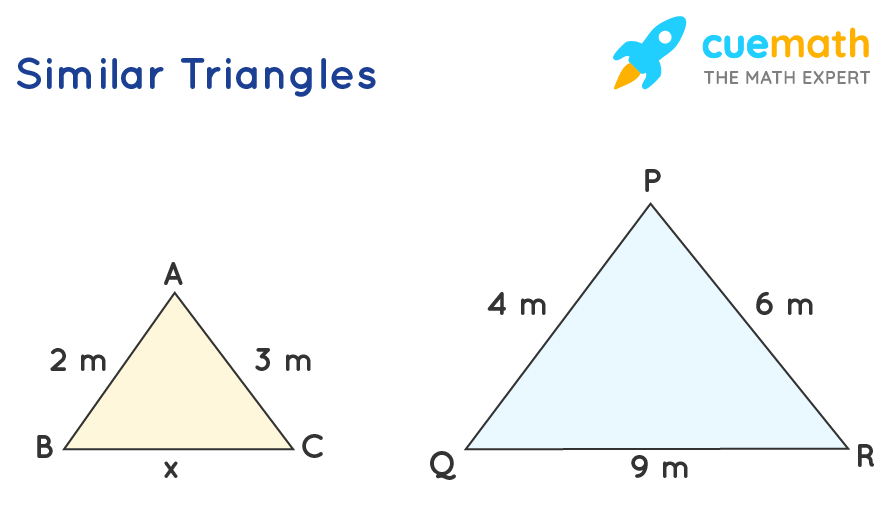# The Missing Side Length

## Question: Use a proportion to find the missing side length in the following similar figures## Answer: The length of the missing side is 4.5m

Two triangles are said to be similar if their corresponding angles are equal and corresponding sides are in the same ratio.

## Explanation:

From the given figure we can see that the sides of the two triangles are in the same proportion as they are similar triangles

AB/PQ = 2/4 = 1/2

AC/PR = 3/6 = 1/2

Thus, AB/PQ = AC/PR = 1/2

Thus, the ratio of the third side will also be in the same proportion i.e., BC/QR = 1/2

=> x/9 = 1/2

=> x = 9/2 = 4.5